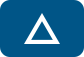## Error message

• Deprecated function: Methods with the same name as their class will not be constructors in a future version of PHP; ctools_context has a deprecated constructor in require_once() (line 127 of /home/tusa/public_html/sites/all/modules/ctools/ctools.module).
• Deprecated function: Methods with the same name as their class will not be constructors in a future version of PHP; ctools_context_required has a deprecated constructor in require_once() (line 127 of /home/tusa/public_html/sites/all/modules/ctools/ctools.module).
• Deprecated function: Methods with the same name as their class will not be constructors in a future version of PHP; ctools_context_optional has a deprecated constructor in require_once() (line 127 of /home/tusa/public_html/sites/all/modules/ctools/ctools.module).
• Deprecated function: Methods with the same name as their class will not be constructors in a future version of PHP; panels_cache_object has a deprecated constructor in require_once() (line 127 of /home/tusa/public_html/sites/all/modules/ctools/ctools.module).

# Free Geometry WorksheetsWe have a great collection of 100% Free Geometry Worksheets with answer keys for use by teachers, students, and homeschool parents. Most worksheets contain an answer key and are formatted for fast and easy printing.

All of our Geometry Worksheets and printables are free for classroom and educational use. They are perfect for students needing some extra geometry practice, teachers for classwork or homework assignments, and homeschool parents for a source of free geometry resources.

Feel free to browse our collection of geometry printables below and print out the ones corresponding to the section or topic you are working on. We have worksheets covering geometry topics from proofs and inductive reasoning to area and circumference, so you are sure to find a suitable worksheet.

You can also browse Geometry Worksheets by Topic

In this free geometry worksheet, students must use formulas for volume to find the volume of objects such as cubes, cylinders, spheres, and pyramids
Worksheet (Geometry)
Problems: 17
In this free worksheet, students must find the area of plane figures such as squares, rectangles, circles, and triangles.
Worksheet (Geometry)
Problems: 16
In this free math worksheet, students must solve problems on geometric progressions. Problems require students to identify terms of geometric progressions and geometric series, to find missing...
Worksheet (Geometry)
Problems: 12
This free geometry worksheet requires the use of the formula for the area of a regular polygon, A = (1/2)ap...where a is the apothem and p is the perimeter of the polygon.
Worksheet (Geometry)
Problems: 10
Problems require students to identify and write biconditional statements (an "if-then" statement that is true in both directions). Students must also identify and explain good definitions.
Worksheet (Geometry)
Problems: 12
This free geometry worksheet contains problems on defining and classifying special quadrilaterals. Students must classify figures as parallelograms, squares, rectangles, rhombuses, trapezoids, and...
Worksheet (Geometry)
Problems: 14
This worksheet contains introductory questions on conditional statements and converses. Students are asked to name and identify the parts of a conditional statement (hypothesis & conclusion), to...
Worksheet (Geometry)
Problems: 11
In this worksheet, students are asked to use congruency statements to identify congruent figures and congruent parts. They are also asked to write congruency statements for pairs of figures, and to...
Worksheet (Geometry)